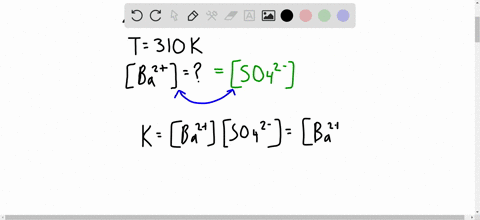Enroll in one of our FREE online STEM bootcamps. Join today and start acing your classes!View Bootcamps### $\mathrm{ABaSO}_{4}$ slurry is ingested before th…

03:52University of California - Los Angeles
Problem 83

# High levels of ozone $\left(\mathrm{O}_{3}\right)$ cause rubber to deteriorate, green plants to turn brown, and many people to have difficulty breathing. (a) Is the formation of $\mathrm{O}_{3}$ from $\mathrm{O}_{2}$ favored at all $T,$ no $T,$ high $T$ or low $T ?$ (b) Calculate $\Delta G^{\circ}$ for this reaction at 298 $\mathrm{K}$ .(c) Calculate $\Delta G$ at 298 $\mathrm{K}$ for this reaction in urban smog where

Check back soon!

## Discussion

You must be signed in to discuss.

## Video Transcript

The question here gives us this reaction, and it wants us to calculate the value of Delta, G and K. So, first of all, to calculate Delta G, we take, um, products minus reactant. So when we calculate this, it should give us a value off negative 198 killer Jules promote. And from here, we can take Delta G is equal to negative, Artie, the natural log of K. And basically, when we plug it in at 298 Kelvin, negative 198 is equal to negative. Negative are which is the ideal gas constant times 298 Kelvin Natural log of K. And when we solve for kay here, this should give us the value of 5.7 times 10 to the power for negative to the power of 34. Rather, And that's the answer to the question here. So K is equal to that value

#### You're viewing a similar answer. To request the exact answer, fill out the form below:

Our educator team will work on creating an answer for you in the next 6 hours.